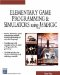# Cos

This command calculates the cosine of an angle. Here is its syntax:

` x = cos(y) `

### Parameters

 x The cosine of angle y. Angle y is measured in radians.

### Notes

Please see Chapter 5 for a more detailed explanation of the cosine function. The cosine is useful when you want to find the horizontal speed of an object that is moving upward at an angle, such as an aircraft climbing.

### Sample Code

The following code sends the angle of roll about the y-axis to the screen, which is controllable with the left and right arrow keys. The program prints the angle and cosine of the angle to the screen.

` oworld = New World(); ocamera = New Camera(oworld); sphere = oworld.CreateSphere(50,50,50,25,25); ocamera.MoveBack(1200); sphere.Walk(); //Loop While(TRUE)  {   z = sphere.getyangle();   Y = cos(z);   SetText("yangle " + z + "cos " + y);   } `Elementary Game Programming & Simulations Using Jamagic (Charles River Media Game Development)
ISBN: 1584502614
EAN: 2147483647
Year: 2002
Pages: 105
Authors: Sergio Perez